<% '下载使用本系统时请将数据库改名。 '不要忘了。改名后请把下面的数据源路径修改一下。 dim db dim connstr dim websql dim webrs dim webname dim url dim mail dim rows dim cel dim img db="mdb/mydatabase.mdb" connstr="DBQ="+server.MapPath(db)+";DefaultDir=;DRIVER={Microsoft Access Driver (*.mdb)}" set conn=server.CreateObject("ADODB.Connection") conn.open connstr websql="select * from config" on error resume next set webrs=server.CreateObject("Adodb.recordset") webrs.open websql,conn,1,1 webname=webrs("webname") url=webrs("url") mail=webrs("mail") rows=webrs("rows") cel=webrs("cel") img=webrs("img") webrs.close set webrs=nothing Sub rsclose() rs.close() set rs=nothing End Sub Sub connclose() conn.close() set conn=nothing End Sub %> <% function UBBCode(strContent) dim re,i 'strContent=encodestr(strContent) 'strContent=funkillWord(strContent) UbbCode=strContent if (instr(strContent,"[")=0 or instr(strContent,"]")=0) and instr(strContent,"http://")=0 then exit function end if Set re=new RegExp re.IgnoreCase =true re.Global=True if instr(1,strContent,"[IMG]",1)>0 then re.Pattern="(\[IMG\])(.[^\[]*)(\[\/IMG\])" strContent=re.Replace(strContent,"500)this.width=500""> ") end if if instr(1,strContent,"[/dir]",1)>0 then re.Pattern="\[DIR=*([0-9]*),*([0-9]*)\](.[^\[]*)\[\/DIR]" strContent=re.Replace(strContent,"") end if if instr(1,strContent,"[/qt]",1)>0 then re.Pattern="\[QT=*([0-9]*),*([0-9]*)\](.[^\[]*)\[\/QT]" strContent=re.Replace(strContent,"") end if if instr(1,strContent,"[/mp]",1)>0 then re.Pattern="\[MP=*([0-9]*),*([0-9]*)\](.[^\[]*)\[\/MP]" strContent=re.Replace(strContent,"") end if if instr(1,strContent,"[/rm]",1)>0 then re.Pattern="\[RM=*([0-9]*),*([0-9]*)\](.[^\[]*)\[\/RM]" strContent=re.Replace(strContent,"
") end if if instr(1,strContent,"[flash]",1)>0 then re.Pattern="(\[FLASH\])(.[^\[]*)(\[\/FLASH\])" strContent= re.Replace(strContent,"[全屏欣赏]
") end if if instr(1,strContent,"[/url]",1)>0 then re.Pattern="(\[URL\])(.[^\[]*)(\[\/URL\])" strContent= re.Replace(strContent,"\$2") re.Pattern="(\[URL=(.[^\]]*)\])(.[^\[]*)(\[\/URL\])" strContent= re.Replace(strContent,"\$3") end if if instr(1,strContent,"[/email]",1)>0 then re.Pattern="(\[EMAIL\])(.[^\[]*)(\[\/EMAIL\])" strContent= re.Replace(strContent,"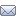\$2") re.Pattern="(\[EMAIL=(.[^\[]*)\])(.[^\[]*)(\[\/EMAIL\])" strContent= re.Replace(strContent,"\$3") end if if instr(1,strContent,"http://",1)>0 then re.Pattern = "^(http://[A-Za-z0-9\./=\?%\-&_~`@':+!]+)" strContent = re.Replace(strContent,"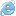\$1") re.Pattern = "(http://[A-Za-z0-9\./=\?%\-&_~`@':+!]+)\$" strContent = re.Replace(strContent,"\$1") re.Pattern = "([^>=""])(http://[A-Za-z0-9\./=\?%\-&_~`@':+!]+)" strContent = re.Replace(strContent,"\$1\$2") end if if instr(1,strContent,"ftp://",1)>0 then re.Pattern = "^(ftp://[A-Za-z0-9\./=\?%\-&_~`@':+!]+)" strContent = re.Replace(strContent,"\$1") re.Pattern = "(ftp://[A-Za-z0-9\./=\?%\-&_~`@':+!]+)\$" strContent = re.Replace(strContent,"\$1") re.Pattern = "([^>=""])(ftp://[A-Za-z0-9\.\/=\?%\-&_~`@':+!]+)" strContent = re.Replace(strContent,"\$1\$2") end if if instr(1,strContent,"rtsp://",1)>0 then re.Pattern = "^(rtsp://[A-Za-z0-9\./=\?%\-&_~`@':+!]+)" strContent = re.Replace(strContent,"\$1") re.Pattern = "(rtsp://[A-Za-z0-9\./=\?%\-&_~`@':+!]+)\$" strContent = re.Replace(strContent,"\$1") re.Pattern = "([^>=""])(rtsp://[A-Za-z0-9\.\/=\?%\-&_~`@':+!]+)" strContent = re.Replace(strContent,"\$1\$2") end if if instr(1,strContent,"mms://",1)>0 then re.Pattern = "^(mms://[A-Za-z0-9\./=\?%\-&_~`@':+!]+)" strContent = re.Replace(strContent,"\$1") re.Pattern = "(mms://[A-Za-z0-9\./=\?%\-&_~`@':+!]+)\$" strContent = re.Replace(strContent,"\$1") re.Pattern = "([^>=""])(mms://[A-Za-z0-9\.\/=\?%\-&_~`@':+!]+)" strContent = re.Replace(strContent,"\$1\$2") end if 're.Pattern="(\[HTML\])(.+?)(\[\/HTML\])" 'strContent=re.Replace(strContent,"
 以下内容为程序代码:\$2
") if instr(1,strContent,"[/color]",1)>0 then re.Pattern="(\[color=(.[^\[]*)\])(.[^\[]*)(\[\/color\])" strContent=re.Replace(strContent,"\$3") end if if instr(1,strContent,"[/face]",1)>0 then re.Pattern="(\[face=(.[^\[]*)\])(.[^\[]*)(\[\/face\])" strContent=re.Replace(strContent,"\$3") end if if instr(1,strContent,"[/align]",1)>0 then re.Pattern="(\[align=(.[^\[]*)\])(.[^\[]*)(\[\/align\])" strContent=re.Replace(strContent,"
\$3
") end if if instr(1,strContent,"[/quote]",1)>0 then re.Pattern="(\[QUOTE\])(.[^\[]*)(\[\/QUOTE\])" strContent=re.Replace(strContent,"
 \$2

") end if if instr(1,strContent,"[/fly]",1)>0 then re.Pattern="(\[fly\])(.[^\[]*)(\[\/fly\])" strContent=re.Replace(strContent,"\$2") end if if instr(1,strContent,"[/move]",1)>0 then re.Pattern="(\[move\])(.[^\[]*)(\[\/move\])" strContent=re.Replace(strContent,"\$2") end if if instr(1,strContent,"[/glow]",1)>0 then re.Pattern="\[GLOW=*([0-9]*),*(#*[a-z0-9]*),*([0-9]*)\](.[^\[]*)\[\/GLOW]" strContent=re.Replace(strContent,"\$4
") end if if instr(1,strContent,"[/i]",1)>0 then re.Pattern="(\[i\])(.[^\[]*)(\[\/i\])" strContent=re.Replace(strContent,"\$2") end if if instr(1,strContent,"[/u]",1)>0 then re.Pattern="(\[u\])(.[^\[]*)(\[\/u\])" strContent=re.Replace(strContent,"\$2") end if if instr(1,strContent,"[/b]",1)>0 then re.Pattern="(\[b\])(.[^\[]*)(\[\/b\])" strContent=re.Replace(strContent,"\$2") end if if instr(1,strContent,"[/size]",1)>0 then re.Pattern="(\[size=1\])(.[^\[]*)(\[\/size\])" strContent=re.Replace(strContent,"\$2") re.Pattern="(\[size=2\])(.[^\[]*)(\[\/size\])" strContent=re.Replace(strContent,"\$2") re.Pattern="(\[size=3\])(.[^\[]*)(\[\/size\])" strContent=re.Replace(strContent,"\$2") re.Pattern="(\[size=4\])(.[^\[]*)(\[\/size\])" strContent=re.Replace(strContent,"\$2") end if if instr(1,strContent,"[/center]",1)>0 then re.Pattern="(\[center\])(.[^\[]*)(\[\/center\])" strContent=re.Replace(strContent,"
\$2
") end if if instr(1,strContent,"[/list]",1)>0 then strContent = doCode(strContent, "[list]", "[/list]", "
", "
") strContent = doCode(strContent, "[list=1]", "[/list]", "
", "
") strContent = doCode(strContent, "[list=a]", "[/list]", "
", "
") end if if instr(1,strContent,"[/*]",1)>0 then strContent = doCode(strContent, "[*]", "[/*]", "
• ", "
• ") end if if instr(1,strContent,"[/code]",1)>0 then strContent = doCode(strContent, "[code]", "[/code]", "
`", "`
") end if for i=0 to 31 re.Pattern="(\[em"&i&"\])" strContent=re.Replace(strContent,"") next set re=Nothing UBBCode=strContent end function function doCode(fString, fOTag, fCTag, fROTag, fRCTag) dim fOTagPos, fCTagPos fOTagPos = Instr(1, fString, fOTag, 1) fCTagPos = Instr(1, fString, fCTag, 1) while (fCTagPos > 0 and fOTagPos > 0) fString = replace(fString, fOTag, fROTag, 1, 1, 1) fString = replace(fString, fCTag, fRCTag, 1,1, 1) fOTagPos = Instr(1, fString, fOTag, 1) fCTagPos = Instr(1, fString, fCTag, 1) wend doCode = fString end function %> Clocks for sale,Customelized Clock,Chem Productions for sale,Pecipitated barium sulfate,Sodium sulfide with low ferric for sale, Goodtime clock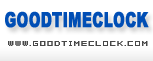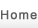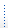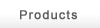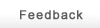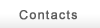Grandpa　ClockCuckoo　ClockMantal　ClockCurioWall　ClockChem products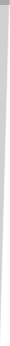· Goodtime clock (Yantai) Co., Ltd.is located in the birthplace of Chinese clock-Yantai.There are more than 200 employees in the factory,50 among which are technicians. We imported advanced equipments and techniques, adopting modern and scientific ways to produce various kinds of mechanical & quartz wooden grandfather clock, mantel clock, wall clock, cuckoo clock and wooden furniture,etc.over 300 types,and the yearly output over 50,000 pieces. We set up special designing department,and there are over 50 new types on sale every year. On the basis of good start, all the products of our company are warmly welcome worldwide, and enjoy good sales in over 50 countries and regions from North and South America, Europe, Middle East, Japan, Africa and Australia. · We insist our motto of “Quality first, Reputation above all”, and supply our customers with prompt delivery and good after-sale service. We go to attend the Canton Fair and the East-China fair every year and negotiate with our customer face to face. We hereby sincerely hope to enter into business relationship with you. Main Products: Grandfather Clock, Wall Clock, Table Clock, Cuckoo Clock and other Wooden Products Materials: Case of Clock: Chinese Linden, Ash, Oak and Chinese Catalpa. Movement:31-day brass mechanical movement made in China, Quartz movement made in China, 8-rod or 12-rod movement made in Germany, or Quartz movement made in Japan.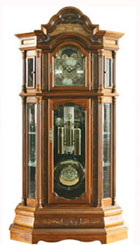Grandpa　Clock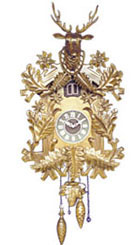Cuckoo　Clock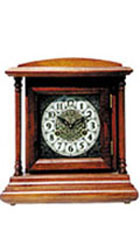Mantal　Clock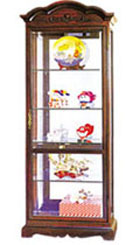Curio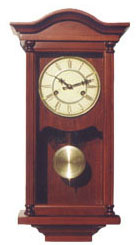Wall　Clock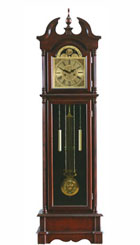Grandpa　Clock
 All Copyright by Goodtime Clock (YanTai)Co.,Ltd 　TEL：86-535-6647900　86-535-6647189 　FAX：86-535-6647095 　Count： ADD:6F/NO.80 DAHAIYANG ROAD,YANTAI SHANDONG CHINA 　　E-mail: whq@public.ytptt.sd.cn 　　鲁ICP备10035068号-1